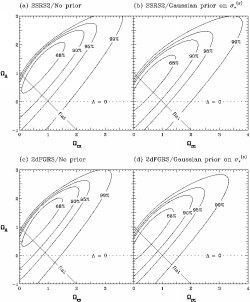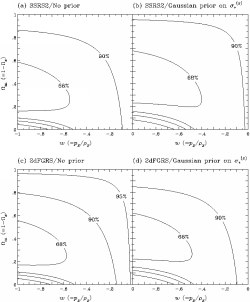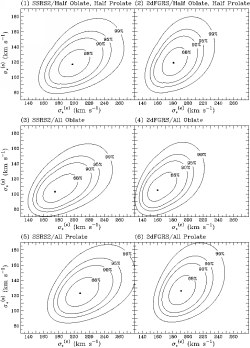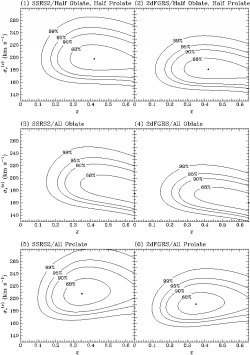Model-fitting and Numerical Simulation

The data and figures on this page are described in K.-H. Chae, The Cosmic Lens All-Sky Survey: statistical strong lensing, cosmological parameters, and global properties of galaxy populations. You can get a postscript preprint from Astro-ph.

Each data file contains a grid of Delta(chi-squared) values on a 2-D or 3-D parameter (sub)space. Here chi-squared = -2 ln(Likelihood). The first column of each file is the array of Delta(chi-squared) values. Please ignore the other columns if present.

Since a multi-dimensional grid was written into a column using a nested loop, the data column has to be read using the same nested loop. I will use letters I, J, and K as indices from the outer to the inner loop. In other words, J runs within I (and K runs within J). Each index corresponds to an axis (of a parameter space) representing an astrophysical quantity.

2-D grids

All the 2-D grid are shown on Figures 6, 7, 8 and 9 of astro-ph/0211244. At each grid point of each parameter plane, the rest of the free parameters were varied to minimize the chi-squared.
 Figure 6I: runs from 0 to 200, corresponds to Omega_matter of [0, 4] J: runs from 0 to 200, corresponds to Omega_Lambda of [-1, 3] Figure 7I: runs from 0 to 100, corresponds to w(equation of state) of [-1, 0] J: runs from 0 to 100, corresponds to Omega_matter of [0, 1] Figure 8I: runs from 0 to 150, corresponds to sigma_*^(e) of [130, 280] in units of km/s J: runs from 0 to 120, corresponds to sigma_*^(s) of [70, 190] in units of km/s Figure 9I: runs from 0 to 60, corresponds to ellipticity of [0.05, 0.65] J: runs from 0 to 150, corresponds to sigma_*^(e) of [130, 280]

3-D grids

The axes of the 3-D space are Omega_matter, Omega_Lambda, & sigma_*^(e). The other two free parameters [i.e. ellipticity & sigma_*^(s)] are held fixed at their global maximum likelihood estimates. The files are gzipped to reduce their size.

Each 3-D grid can be regarded as the sigma_*^(e) axis added to the Omega_matter-Omega_Lambda plane. Thus, CLASS_3Dgrid_x.dat corresponds to the extension of OmLam_CLASS_profgrid_x.dat (where x=a, b, c, d) shown on Fig. 6.

I: runs from 0 to 200 corresponds to Omega_matter of [0, 4]

J: runs from 0 to 200, corresponds to Omega_Lambda of [-1, 3]

K: runs from 0 to 200, corresponds to sigma_*^(e) of [100, 300]